Science, Maths & Technology

### Become an OU student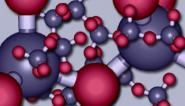The molecular world

Start this free course now. Just create an account and sign in. Enrol and complete the course for a free statement of participation or digital badge if available.

# 1.5 Non-molecular substances

Non-molecular substances defy attempts to pick out discrete molecules from their structures. One example is common salt, NaCl, which is built up from the tiny cubes shown in Figure 10a. Look first at the sodium at the centre of the cube.

## Question 7

What kind of atom is closest to the sodium, and how many of them are there?

The sodium is surrounded by six chlorines at the centres of the cube faces.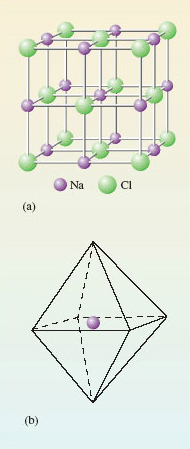Figure 10 (a) Structure of common salt or sodium chloride; (b) a regular octahedron whose corners represent the positions of the chlorines around each sodium

The six chlorines lie at the corners of a three-dimensional figure called a regular octahedron (a solid figure with eight faces; see Figure 10b). The formula NaCl for sodium chloride is an empirical formula: it merely tells us that in sodium chloride there are equal numbers of sodiums and chlorines. This condition is automatically fulfilled when many cubes of the Figure 10a type are joined through their faces. But Figure 10 provides no evidence that NaCl is the molecular formula of sodium chloride. Indeed, quite the opposite, because the six chlorines around the sodium in Figure 10a all lie the same distance away. There are no grounds for singling out just one of them and coupling it with the sodium as an NaCl molecule. There is no evidence of discrete NaCl molecules in the solid; NaCl is a non-molecular compound, and the concept of a 'molecular formula' is not appropriate in solid NaCl.

Similar considerations apply to silicon dioxide or silica, SiO2. This is the main component of sand, and it has the same type of empirical formula as carbon dioxide. In solid carbon dioxide, two of the oxygen atoms around each carbon were much closer than the others, so we could identify a CO2 molecule. However, in silica (Figure 11), each silicon atom sits at the centre of a tetrahedron of oxygen atoms: the silicon is surrounded by four oxygen atoms, all at the same distance of 162 pm. There is no evidence of discrete SiO2 molecules.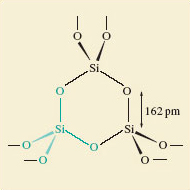Figure 11 The structure of silica, SiO2, in the form of quartz. One SiO4 tetrahedron is highlighted in green.

Most of the chemical elements are non-molecular substances. Figure 12 shows the environment of each atom in diamond and metallic aluminium. In diamond (Figure 12a), there are four surrounding carbon atoms at the corners of a regular tetrahedron, and the C—C distance is 154 pm. In aluminium (Figure 12b), there are twelve surrounding aluminium atoms, and the Al—Al distance is 286 pm. There is no justification for dividing the structure up into molecules containing two or more atoms. Any such 'molecule' extends throughout a crystal of the substance, and its formula will vary with the crystal size. For this reason, the phrase extended structure is sometimes used to describe non-molecular substances.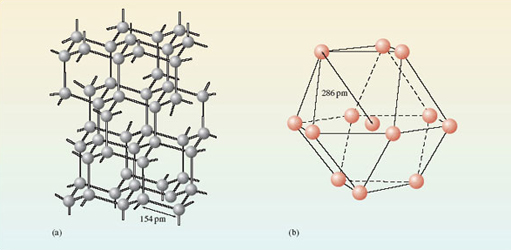Figure 12 The environment of each atom in (a) the diamond form of the element carbon; (b) the metallic element aluminium. Both substances are non-molecular, and have extended structures.

In Figures 12a and 12b, the extension occurs in three dimensions, but it may sometimes reveal itself in only one or two. Figure 13 shows the structure of graphite, the form of carbon used in pencil 'leads'. There are regular hexagons of carbon atoms arranged in parallel sheets. Within the sheets, the C—C distance is only 142 pm, but the shortest distances between the sheets is 340 pm.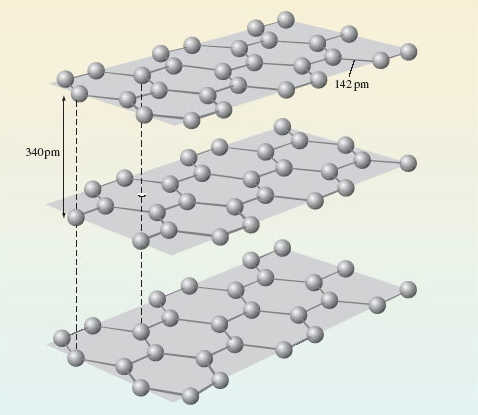Figure 13 The structure of the graphite form of the element carbon

## Question 8

If one regards a single crystal of graphite as an extended structure, does the extension occur in one, two or three dimensions?